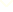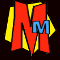Welcome to our PRIME COUNTER!             If the numbers or flashlines are behaving oddly, please click RESET to synchronise them.Please scroll down to read this page.WHAT IS A PRIME NUMBER? A prime number will only divide exactly by itself and the number 1. 7 IS prime because it only divides by 7 and 1. 8 is NOT prime because it divides by 1,2,4 and 8. Numbers that are not prime are called COMPOSITE numbers. There's more about prime numbers and a special prime calculator on our prime numbers page.

WHAT DOES THE 1-100 GRID SHOW? The green numbers are prime . The grey numbers are composite. Nobody is sure whether 1 is prime or not!

WHAT IS THE COUNTER DEMONSTRATING? All the grey numbers between 4 and 100 will divide by at least one of the primes 2,3,5 or 7. As the counter goes up, the primes flash when they will divide in.

WHAT DOES IT MEAN WHEN TWO PRIMES FLASH TOGETHER? Watch what happens whenever the counter ends with "0". You'll see that the 2 and the 5 flash together. This is because 2x5=10. Likewise 2 and 3 will flash together for any number that divides by 6 because 2x3=6.

WHAT DOES IT MEAN WHEN THREE PRIMES FLASH TOGETHER? Watch what happens when the counter reaches 30. You'll see the 2,3 and 5 all flash. This is because 2x3x5=30. These three will always flash together for any number that divides by 30. In the same way, 2x5x7=70, so the 2,5 and 7 will all flash together for any number that divides by 70.

WHEN WILL THE 2,3,5 AND 7 ALL FLASH TOGETHER? 2x3x5x7=210, so they will all flash at 210. Then they'll all flash again at any number dividing by 210 including 420, 630 and 840.

WHAT ARE THE PRIMES 11-31 FOR? The counter continues up to 999 before it resets. All the composite numbers up to 999 will divide by at least one of the primes up to 31. If the counter shows a number and none of the primes is flashing, then that number is itself prime. The lowest primes after 100 are 101, 103, 107, 109, 113 then they are all composite until 127, 131, 137 ... The higher the counter goes, the rarer the primes become.

HOW LONG WOULD YOU HAVE TO WAIT FOR ALL THE ELEVEN FEATURED PRIMES TO FLASH AT ONCE? The eleven primes all flash together at 000, but after that the next number would be: 2x3x5x7x11x13x17x19x23x29x31 = 200,560,490,130. Our counter changes twice every second, so if it didn't reset at 999, to reach this number would take nearly 3178 years!

IS THERE A PATTERN TO PRIMES? No! People have spent thousands of years trying to make formulas to predict prime numbers but they have all failed.

IS THERE A BIGGEST POSSIBLE PRIME NUMBER? No. It's easy to show that prime numbers go on for ever, and super computers are always looking for bigger and bigger primes. There's more about primes and the strange things they do in Numbers the Key to the Universe.The Prime Numbers page with a prime calculator and magic trick!

Tricks and Games

The Murderous Maths main index page